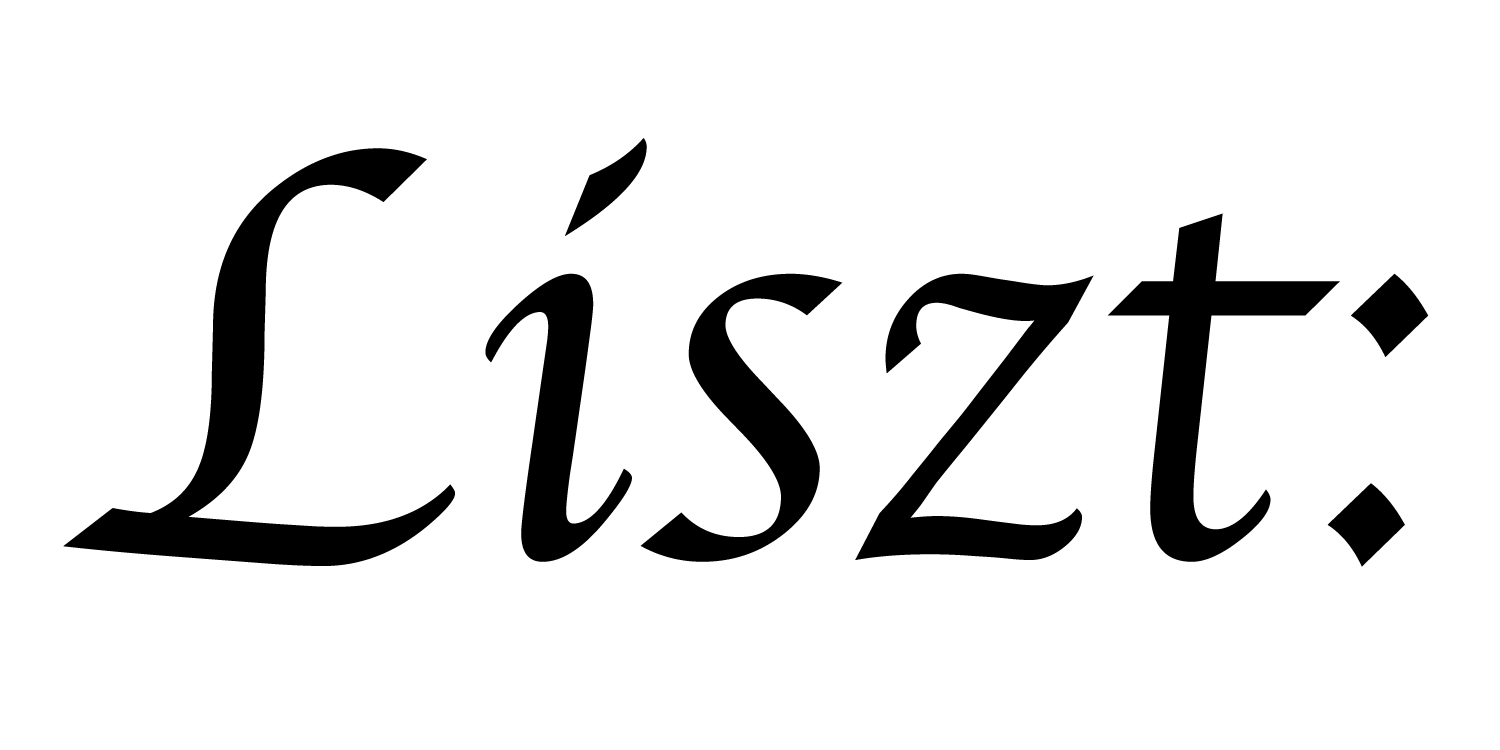# 14: Join Tables

Somewhat surprisingly, key-fields together with group-by/query-loops are sufficient to express arbitrary graph connectivity patterns. To do so, we use a well known trick from databases, called a join table. Unlike the relational tables we’ve been declaring up to this point, join tables don’t represent a particular set of objects. Instead, they represent a relationship between two different other sets of objects. As an example, we’ll load in a standard triangle-mesh and augment it with a way to get all of the triangles touching a given vertex.

``````import 'ebb'
local L = require 'ebblib'

local ioOff = require 'ebb.domains.ioOff'
local PN    = require 'ebb.lib.pathname'
local mesh  = ioOff.LoadTrimesh( PN.scriptdir() .. 'bunny.off' )

local vdb   = require('ebb.lib.vdb')
``````

Our program starts in the usual way.

``````local v_triples       = mesh.triangles.v:Dump({})
local tri_ids, v_ids  = {}, {}
for k=0,mesh.triangles:Size()-1 do
tri_ids[ 3*k + 1 ] = k
tri_ids[ 3*k + 2 ] = k
tri_ids[ 3*k + 3 ] = k
local triple = v_triples[k+1]
v_ids[ 3*k + 1 ] = triple
v_ids[ 3*k + 2 ] = triple
v_ids[ 3*k + 3 ] = triple
end

local triangles_of_vertex = L.NewRelation {
name = "triangles_of_vertex",
size = #tri_ids,
}
``````

This is the join-table. It contains one row for each triangle-vertex pair that are in contact. This table now explicitly represents the connection between the triangles and vertices.

``````triangles_of_vertex:GroupBy('v')
``````

When we group this join-table by the vertices, we prepare it so that we can quickly query for all the rows with a given vertex. This will allow us to iterate over all the triangles attached to a given vertex. If we want to also access this table by the vertices, we’ll have to make a second copy that we can group a second way.

Rather than simulate, we’re going to visualize the dual-area around the vertices.

``````mesh.vertices:NewField('dual_area', L.double):Load(0.0)

local ebb compute_area ( t : mesh.triangles )
var e01 = t.v.pos - t.v.pos
var e02 = t.v.pos - t.v.pos

t.area = L.length( L.cross(e01, e02) )
end
mesh.triangles:foreach(compute_area)
``````

We compute triangle areas the standard way.

``````local ebb compute_dual_area ( v : mesh.vertices )
for t in L.Where(triangles_of_vertex.v, v).tri do
v.dual_area += t.area
end
v.dual_area = v.dual_area / 3.0
end
mesh.vertices:foreach(compute_dual_area)
``````

Dual areas are computed from the vertices using the triangles_of_vertex join-table we set up. This is a query loop like we saw in the last tutorial, but with a slight modification. After the `L.Where(...)` we have a post-fix `.tri` as if we were accessing a field. In order to simplify the use of join-tables, Ebb allows for this special bit of syntax sugar.

``````local ebb visualize ( v : mesh.vertices )
var a = L.fmin( L.fmax( v.dual_area * 2.0 - 0.5, 0.0 ), 1.0 )
vdb.color({ 0.5-a, 0.5 * a + 0.5, 0.5 * a + 0.5 })
vdb.point(v.pos)
end
mesh.vertices:foreach(visualize)
``````

Finally, we visualize the vertex area using a color encoding.

a part of the Liszt project and PSAAP II center at Stanford University